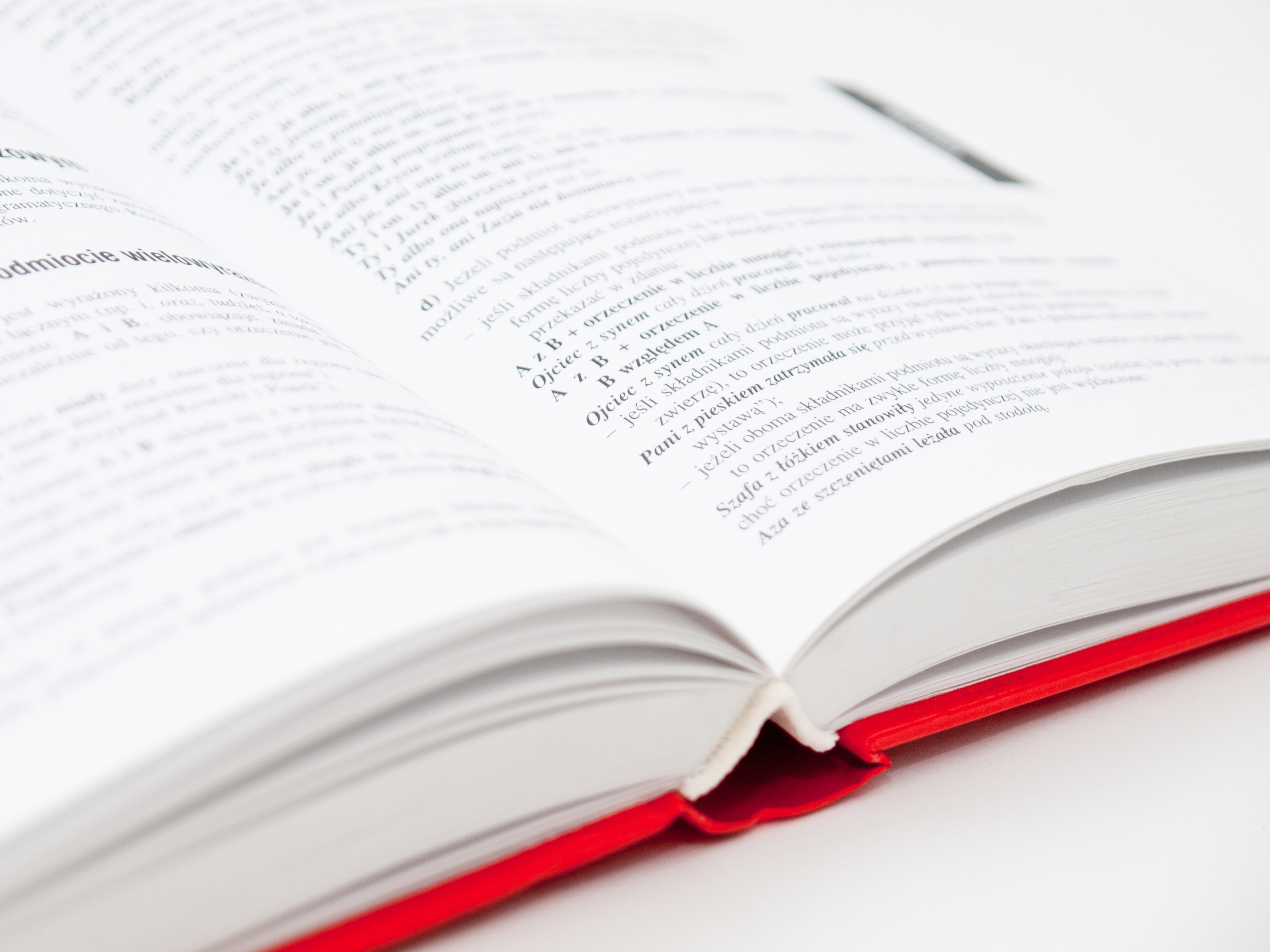# Physics Numericals class 9 (CBSE/ICSE)–problem set2

Here we present another set of physics numerical for class 9. You can also get the solutions for this set of physics numerical for class 9. The Links to the solution are given beside the problems.

You can try first try and then check the solutions. Or you can also directly refer to the solutions of these numerical problems and try to grab the tricks and steps of problem-solving.

You should solve the first set of physics problems for class 9 as well.

## Physics numericals class 9

Set 2

1) A force acts for 10 s on a stationary body of mass 100 kg after which the force ceases to act. The body moves through a distance of 100 m in the next 5 s. Calculate (i) the velocity acquired by the body, (ii) the acceleration produced by the force, and (iii) the magnitude of the force.

2) A force acts for 0.1 sec on a body of mass 2 kg initially at rest. The force is then withdrawn and the body moves with a velocity of 2 m/s. Find the magnitude of the force.

3)A cricket ball of mass 100 g moving at a speed of 30 m/s is brought to rest by a player in 0.03 s. find the average force applied by the player.

4)A body of mass 500 g, initially at rest, is acted upon by a force that causes it to move a distance of 4 meters in 2 sec. Calculate the force applied.

## more numerical problems

5)A force causes an acceleration of 10 m/s^2 in a body of mass 500 g. What acceleration would be caused by the same force in a body of mass 5 kg.

6)A bullet of mass 50 g moving with an initial velocity of 100 m/s, strikes a wooden block and comes to rest after penetrating a distance of 2 cm in it.
Calculate:
(i) Initial momentum of the bullet (ii)final momentum of the bullet (iii) retardation caused by the wooden block, and (iv) resistive force exerted by the wooden block